HomeBlog
Published on November 11, 2017

# Building a Connected Ammeter with Arduino

As I continue my electronic experiments, I wanted to measure the current used by ESP8266 at its different stages. I didn’t wanna just look at numbers, but visualize the current via graphs. The current range I wanted to measure is from 120mA to 30uA. At the end, I wanted a graph like this: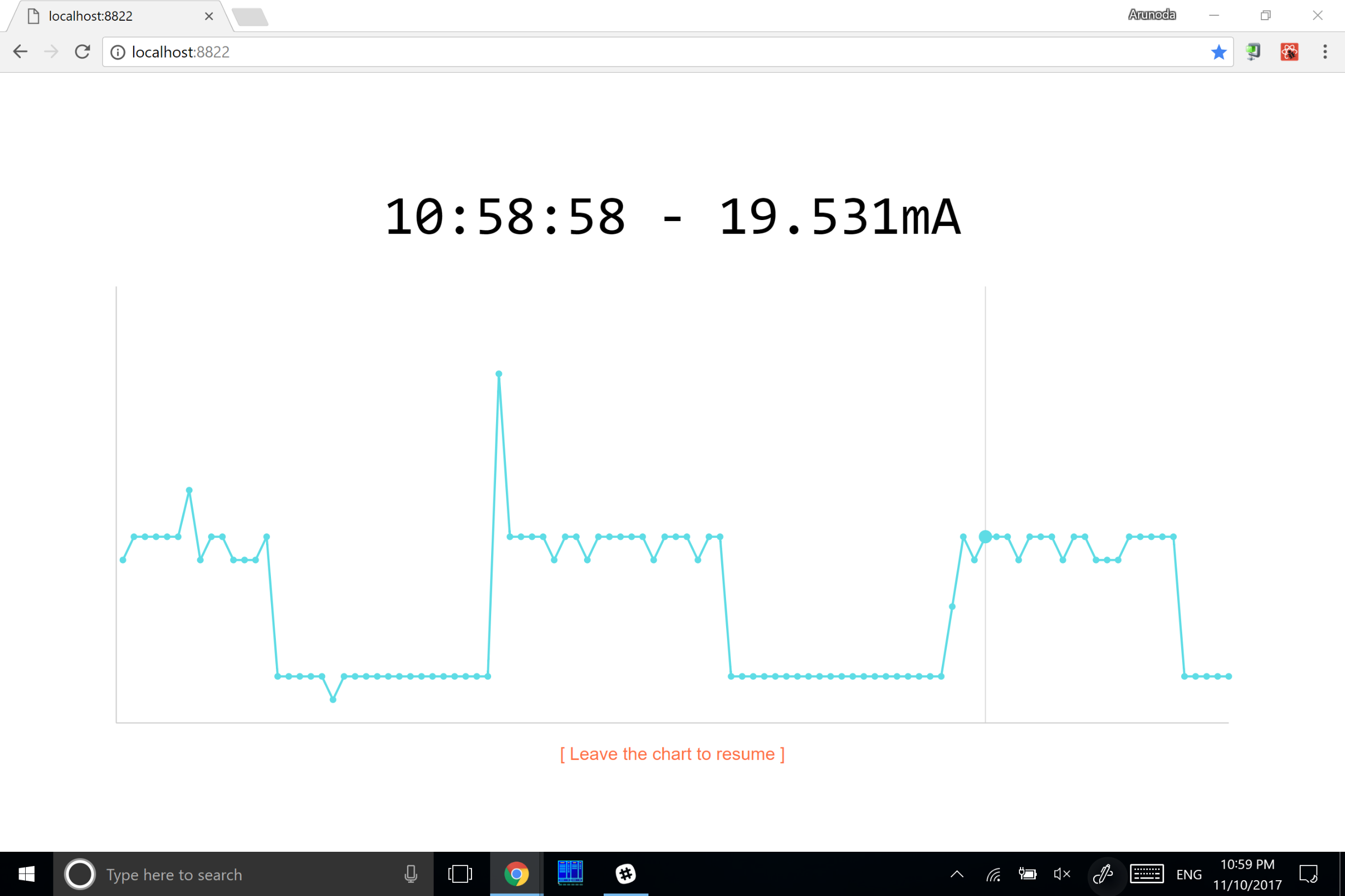## Measuring the voltage with Arduino

Arduino has a set of pins which we can use to measure the voltage. We can measure up to 5V in a range of 1024 values. Therefore, the voltage resolution is basically around 5 mv (5000mv/1024).
This is my Arduino setup: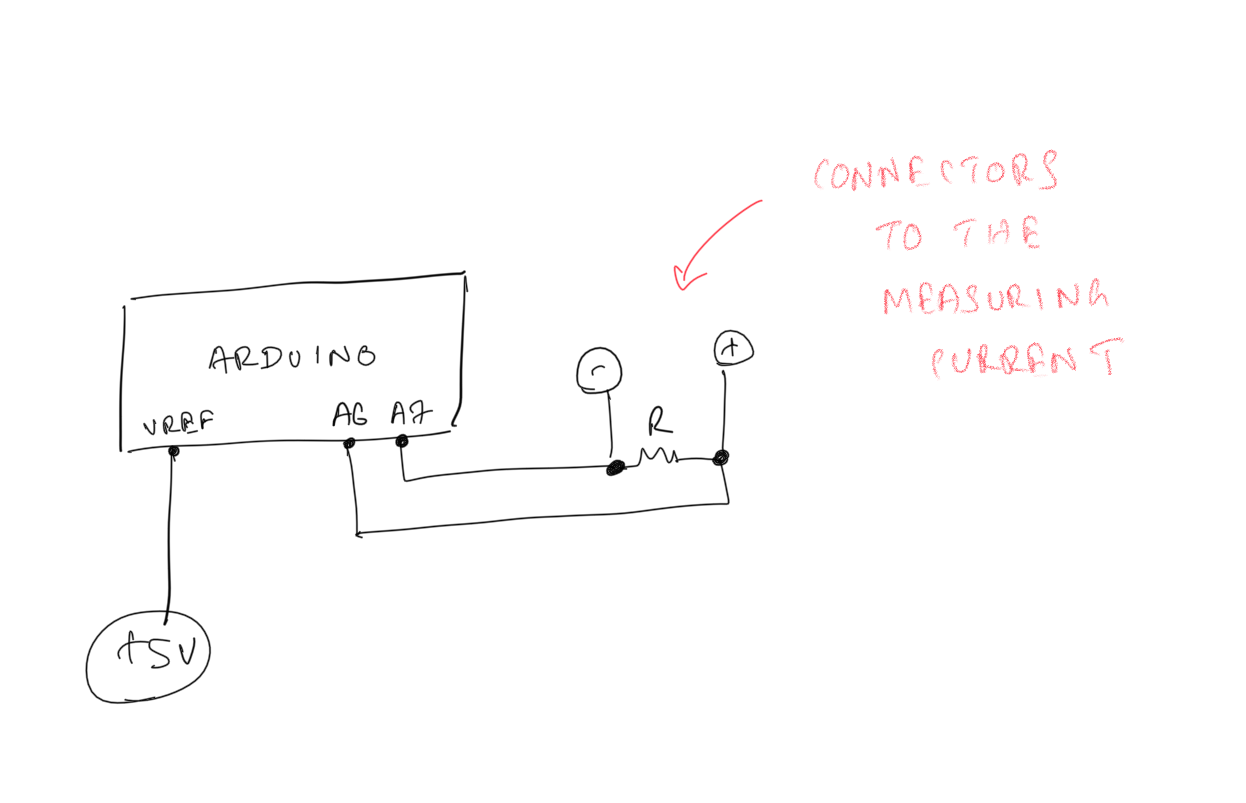Here's the code:
``````void setup() {
Serial.begin(9600);
}

float toVolt(int val) {
return (val/1024.0) * 5.0;
}

void loop() {

float i = ((a6 - a7) / 100.0) * 1000000;
Serial.println(String(i) + "uA");

delay(250);
}``````
Basically, I'm measuring the voltage between the resistor and applying I=V/R to calculate the current.
It works well. Initially, the accuracy was not that precise. However, I was able to bump up the accuracy by attaching a 5v connection to the AREF pin in Arduino. With that, we can tell Arduino to use that voltage as the reference for analogRead.

## Dashboard

As you've noticed in the above Arduino code, I sent measurements to the serial port every 250ms. Then I wrote a simple Next.js app to visualize those measurements.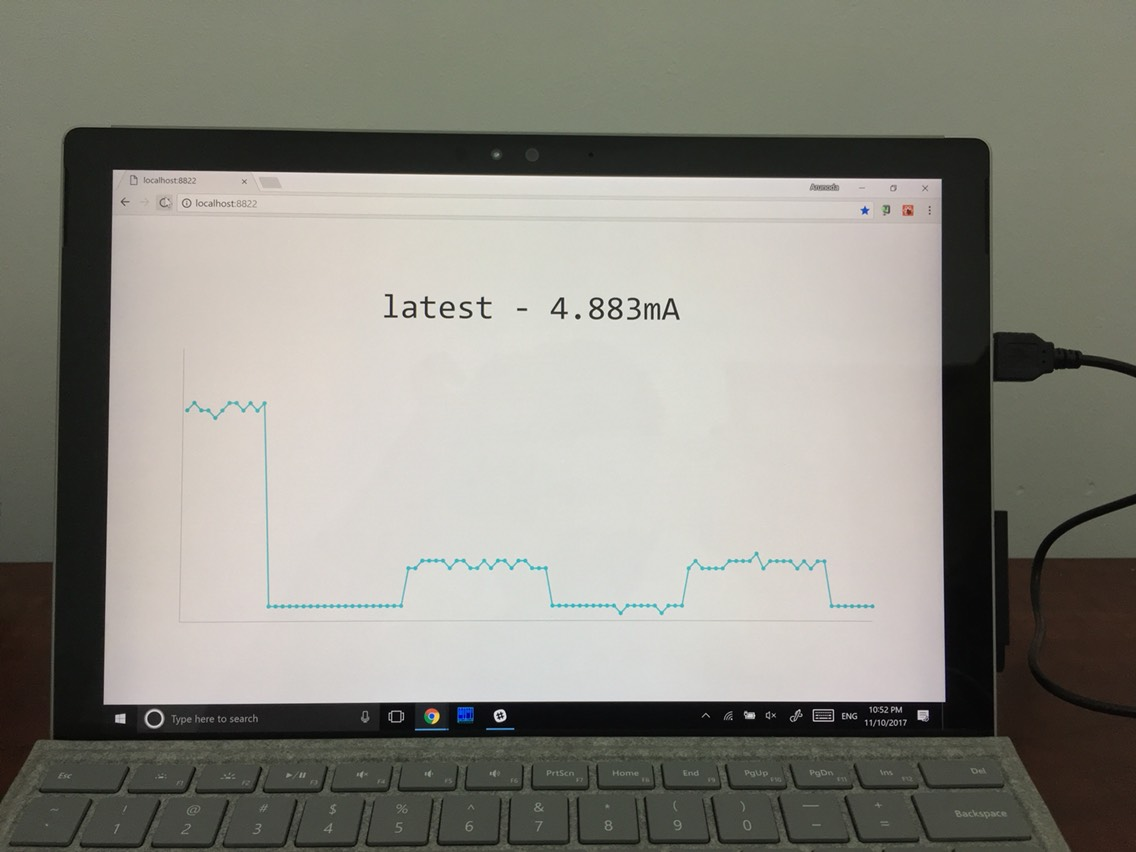It's pretty simple and it achieves what I want.

## Actual Usage

Next, I started using my new ammeter to monitor the current usage of ESP8266. Here's my setup: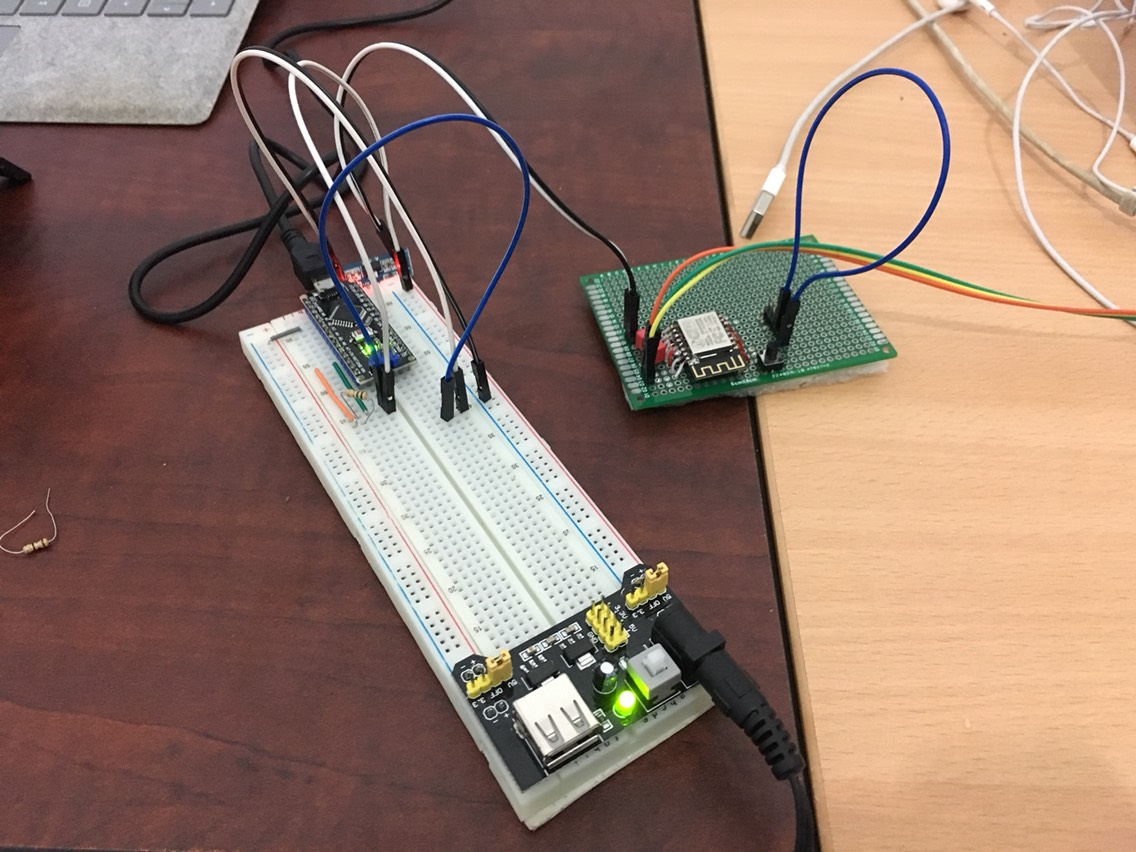Initially, it failed. I used a 100ohms resistor, where I could measure as low as 50uA of current. I came up with that number by applying I=V/R and using V as 5mv (which is the minimal voltage Arduino can measure).
But it didn't work well.
The reason is ESP8266 didn't get enough voltage to power it up. It needs exactly 3.3v. I used a voltage regulator, but the input to that was 5v. The 100ohms resistor takes a considerable amount of voltage. Hence, the input voltage to the 3.3v voltage regulator was less than 3.3v.
I could have used a higher input of voltage like 12v and give it a try, but I didn't have a good 12v input source (or I was lazy to find one).

## Making it Work

I then used a 2ohms resistor and got it working. Then the minimal current I could measure was around 2.5 mA. That was not good enough for me.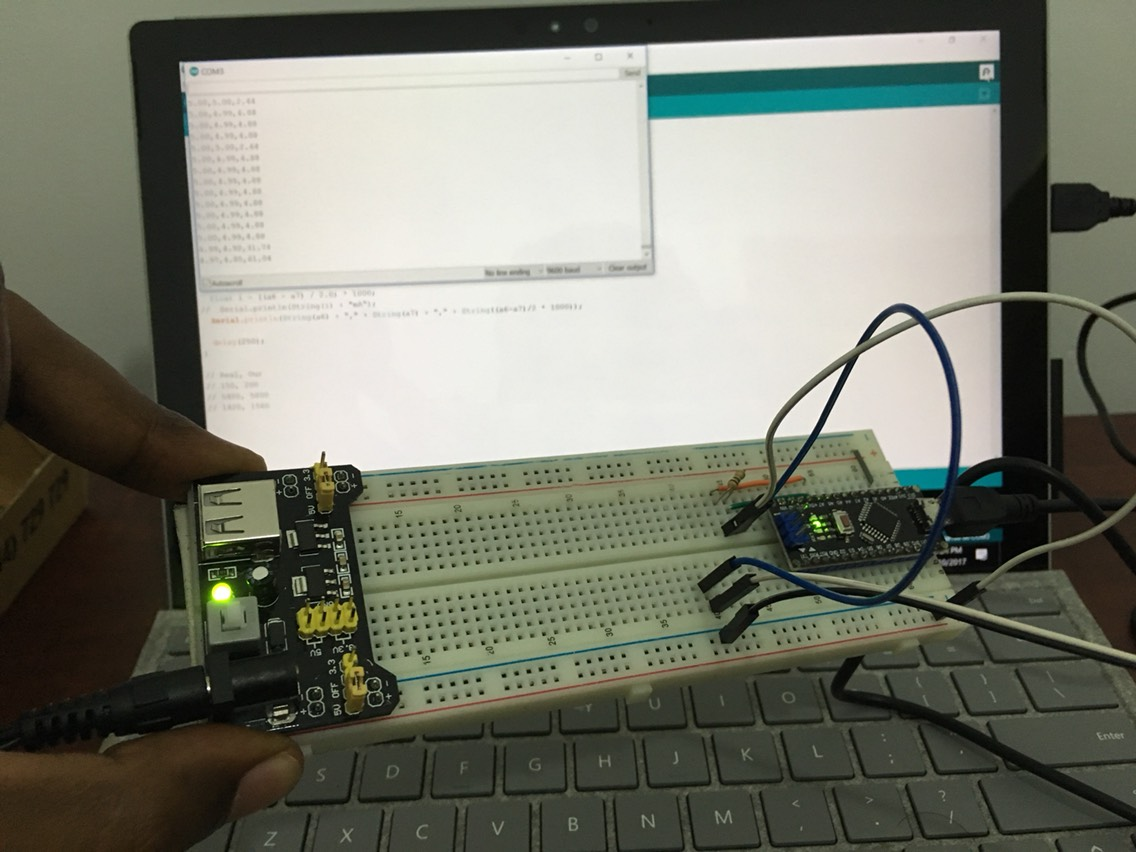## In the End

Basically, Arduino is effective at measuring voltage. But it's not good for measuring very low ranges of current.
However, I was able to get an idea of the current usage of ESP8266 and that's good enough for me (at least for now).
My original idea was to build a connected ammeter with some pretty dashboards. But since I can't measure very low current, I am not continuing this project. Nevertheless, this was a very nice experiment and I learnt a lot.

## Next Step

As you already know, high precision ammeters are very expensive. But I found a cheap ammeter which is good enough in my case.
After I got it, I'd like to test it and connect it my Next.js powered dashboard. Let’s hope it’ll work well.